# 10.7 Parametric equations: graphs  (Page 4/4)

 Page 4 / 4

Graph on the domain $\text{\hspace{0.17em}}\left[-\pi ,0\right],\text{\hspace{0.17em}}$ where $\text{\hspace{0.17em}}a=5\text{\hspace{0.17em}}$ and $\text{\hspace{0.17em}}b=4$ , and include the orientation.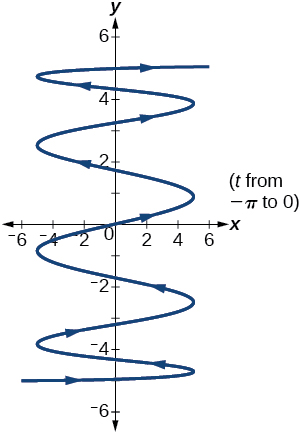If $\text{\hspace{0.17em}}a\text{\hspace{0.17em}}$ is 1 more than $\text{\hspace{0.17em}}b,\text{\hspace{0.17em}}$ describe the effect the values of $\text{\hspace{0.17em}}a\text{\hspace{0.17em}}$ and $\text{\hspace{0.17em}}b\text{\hspace{0.17em}}$ have on the graph of the parametric equations.

Describe the graph if $\text{\hspace{0.17em}}a=100\text{\hspace{0.17em}}$ and $\text{\hspace{0.17em}}b=99.$

There will be 100 back-and-forth motions.

What happens if $\text{\hspace{0.17em}}b\text{\hspace{0.17em}}$ is 1 more than $\text{\hspace{0.17em}}a?\text{\hspace{0.17em}}$ Describe the graph.

If the parametric equations $\text{\hspace{0.17em}}x\left(t\right)={t}^{2}\text{\hspace{0.17em}}$ and $\text{\hspace{0.17em}}y\left(t\right)=6-3t\text{\hspace{0.17em}}$ have the graph of a horizontal parabola opening to the right, what would change the direction of the curve?

Take the opposite of the $\text{\hspace{0.17em}}x\left(t\right)\text{\hspace{0.17em}}$ equation.

For the following exercises, describe the graph of the set of parametric equations.

$x\left(t\right)=-{t}^{2}\text{\hspace{0.17em}}$ and $\text{\hspace{0.17em}}y\left(t\right)\text{\hspace{0.17em}}$ is linear

$y\left(t\right)={t}^{2}\text{\hspace{0.17em}}$ and $\text{\hspace{0.17em}}x\left(t\right)\text{\hspace{0.17em}}$ is linear

The parabola opens up.

$y\left(t\right)=-{t}^{2}\text{\hspace{0.17em}}$ and $\text{\hspace{0.17em}}x\left(t\right)\text{\hspace{0.17em}}$ is linear

Write the parametric equations of a circle with center $\text{\hspace{0.17em}}\left(0,0\right),$ radius 5, and a counterclockwise orientation.

$\left\{\begin{array}{l}x\left(t\right)=5\mathrm{cos}t\\ y\left(t\right)=5\mathrm{sin}t\end{array}$

Write the parametric equations of an ellipse with center $\text{\hspace{0.17em}}\left(0,0\right),$ major axis of length 10, minor axis of length 6, and a counterclockwise orientation.

For the following exercises, use a graphing utility to graph on the window $\text{\hspace{0.17em}}\left[-3,3\right]\text{\hspace{0.17em}}$ by $\text{\hspace{0.17em}}\left[-3,3\right]\text{\hspace{0.17em}}$ on the domain $\text{\hspace{0.17em}}\left[0,2\pi \right)\text{\hspace{0.17em}}$ for the following values of $\text{\hspace{0.17em}}a\text{\hspace{0.17em}}$ and $\text{\hspace{0.17em}}b$ , and include the orientation.

$\left\{\begin{array}{l}x\left(t\right)=\mathrm{sin}\left(at\right)\\ y\left(t\right)=\mathrm{sin}\left(bt\right)\end{array}$

$a=1,b=2$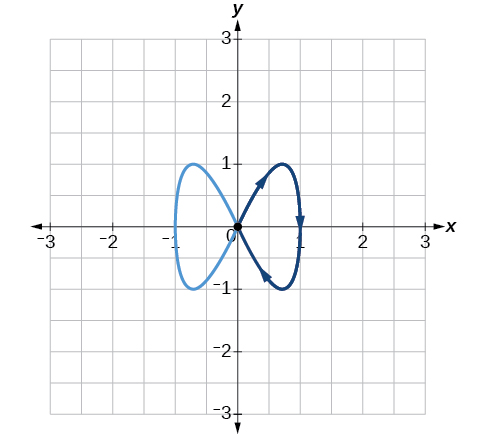$a=2,b=1$

$a=3,b=3$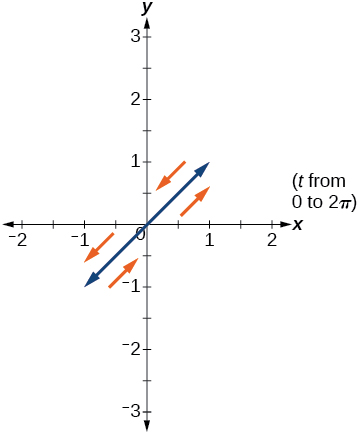$a=5,b=5$

$a=2,b=5$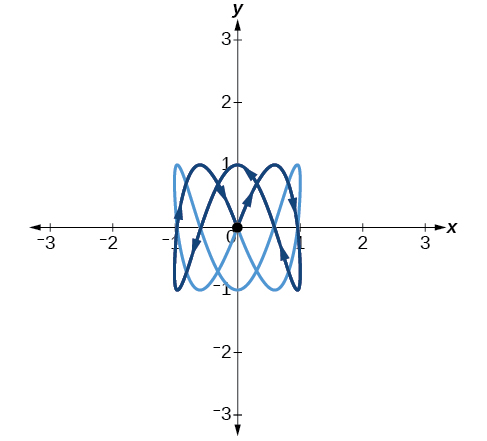$a=5,b=2$

## Technology

For the following exercises, look at the graphs that were created by parametric equations of the form $\text{\hspace{0.17em}}\left\{\begin{array}{l}x\left(t\right)=a\text{cos}\left(bt\right)\hfill \\ y\left(t\right)=c\text{sin}\left(dt\right)\hfill \end{array}.\text{\hspace{0.17em}}$ Use the parametric mode on the graphing calculator to find the values of $a,b,c,$ and $d$ to achieve each graph.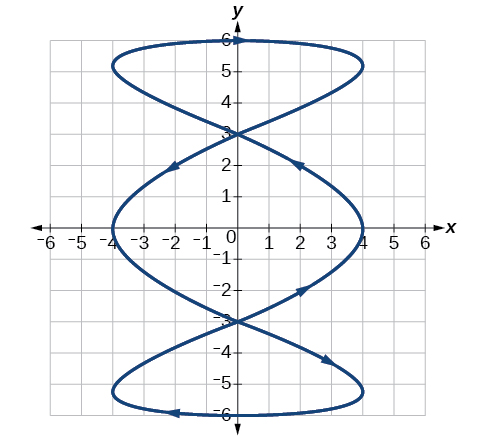$a=4,\text{\hspace{0.17em}}b=3,\text{\hspace{0.17em}}c=6,\text{\hspace{0.17em}}d=1$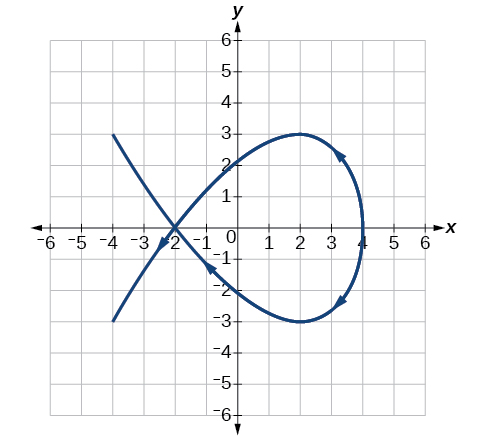$a=4,\text{\hspace{0.17em}}b=2,\text{\hspace{0.17em}}c=3,\text{\hspace{0.17em}}d=3$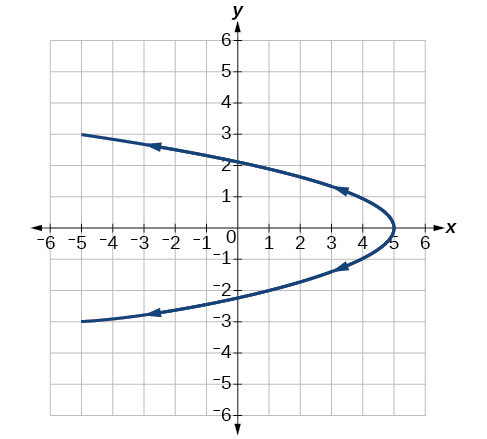For the following exercises, use a graphing utility to graph the given parametric equations.

1. $\left\{\begin{array}{l}x\left(t\right)=\mathrm{cos}t-1\\ y\left(t\right)=\mathrm{sin}t+t\end{array}$
2. $\left\{\begin{array}{l}x\left(t\right)=\mathrm{cos}t+t\\ y\left(t\right)=\mathrm{sin}t-1\end{array}$
3. $\left\{\begin{array}{l}x\left(t\right)=t-\mathrm{sin}t\\ y\left(t\right)=\mathrm{cos}t-1\end{array}$

Graph all three sets of parametric equations on the domain $\text{\hspace{0.17em}}\left[0,\text{\hspace{0.17em}}2\pi \right].$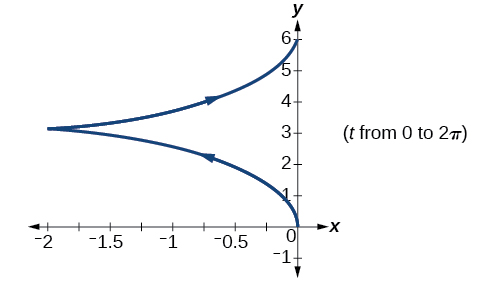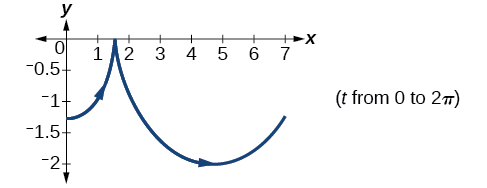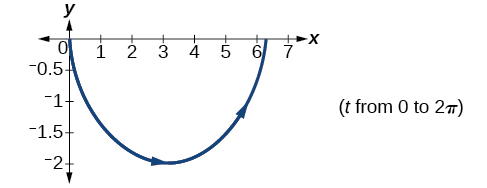Graph all three sets of parametric equations on the domain $\text{\hspace{0.17em}}\left[0,4\pi \right].$

Graph all three sets of parametric equations on the domain $\text{\hspace{0.17em}}\left[-4\pi ,6\pi \right].$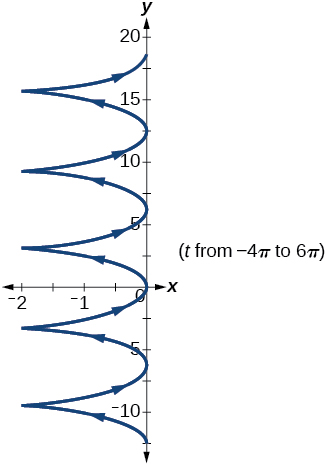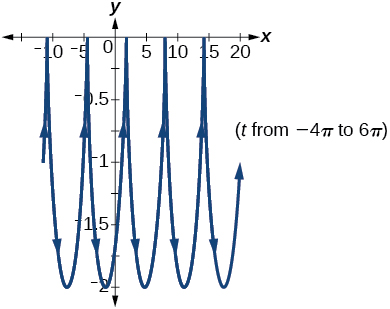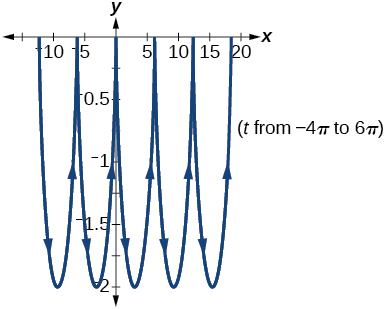The graph of each set of parametric equations appears to “creep” along one of the axes. What controls which axis the graph creeps along?

Explain the effect on the graph of the parametric equation when we switched $\text{\hspace{0.17em}}\mathrm{sin}\text{\hspace{0.17em}}t\text{\hspace{0.17em}}$ and $\text{\hspace{0.17em}}\mathrm{cos}\text{\hspace{0.17em}}t$ .

The $\text{\hspace{0.17em}}y$ -intercept changes.

Explain the effect on the graph of the parametric equation when we changed the domain.

## Extensions

An object is thrown in the air with vertical velocity of 20 ft/s and horizontal velocity of 15 ft/s. The object’s height can be described by the equation $\text{\hspace{0.17em}}y\left(t\right)=-16{t}^{2}+20t$ , while the object moves horizontally with constant velocity 15 ft/s. Write parametric equations for the object’s position, and then eliminate time to write height as a function of horizontal position.

$y\left(x\right)=-16{\left(\frac{x}{15}\right)}^{2}+20\left(\frac{x}{15}\right)$

A skateboarder riding on a level surface at a constant speed of 9 ft/s throws a ball in the air, the height of which can be described by the equation $\text{\hspace{0.17em}}y\left(t\right)=-16{t}^{2}+10t+5.\text{}$ Write parametric equations for the ball’s position, and then eliminate time to write height as a function of horizontal position.

For the following exercises, use this scenario: A dart is thrown upward with an initial velocity of 65 ft/s at an angle of elevation of 52°. Consider the position of the dart at any time $\text{\hspace{0.17em}}t.\text{\hspace{0.17em}}$ Neglect air resistance.

Find parametric equations that model the problem situation.

$\left\{\begin{array}{l}x\left(t\right)=64t\mathrm{cos}\left(52°\right)\\ y\left(t\right)=-16{t}^{2}+64t\mathrm{sin}\left(52°\right)\end{array}$

Find all possible values of $\text{\hspace{0.17em}}x\text{\hspace{0.17em}}$ that represent the situation.

When will the dart hit the ground?

approximately 3.2 seconds

Find the maximum height of the dart.

At what time will the dart reach maximum height?

1.6 seconds

For the following exercises, look at the graphs of each of the four parametric equations. Although they look unusual and beautiful, they are so common that they have names, as indicated in each exercise. Use a graphing utility to graph each on the indicated domain.

An epicycloid: $\text{\hspace{0.17em}}\left\{\begin{array}{l}x\left(t\right)=14\mathrm{cos}\text{\hspace{0.17em}}t-\mathrm{cos}\left(14t\right)\hfill \\ y\left(t\right)=14\mathrm{sin}\text{\hspace{0.17em}}t+\mathrm{sin}\left(14t\right)\hfill \end{array}\text{\hspace{0.17em}}$ on the domain $\text{\hspace{0.17em}}\left[0,2\pi \right]$ .

A hypocycloid: $\left\{\begin{array}{l}x\left(t\right)=6\mathrm{sin}\text{\hspace{0.17em}}t+2\mathrm{sin}\left(6t\right)\hfill \\ y\left(t\right)=6\mathrm{cos}\text{\hspace{0.17em}}t-2\mathrm{cos}\left(6t\right)\hfill \end{array}\text{\hspace{0.17em}}$ on the domain $\text{\hspace{0.17em}}\left[0,2\pi \right]$ .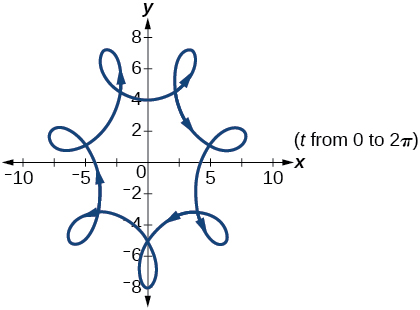A hypotrochoid: $\left\{\begin{array}{l}x\left(t\right)=2\mathrm{sin}\text{\hspace{0.17em}}t+5\mathrm{cos}\left(6t\right)\hfill \\ y\left(t\right)=5\mathrm{cos}\text{\hspace{0.17em}}t-2\mathrm{sin}\left(6t\right)\hfill \end{array}\text{\hspace{0.17em}}$ on the domain $\text{\hspace{0.17em}}\left[0,2\pi \right]$ .

A rose: $\text{\hspace{0.17em}}\left\{\begin{array}{l}x\left(t\right)=5\mathrm{sin}\left(2t\right)\mathrm{sin}t\hfill \\ y\left(t\right)=5\mathrm{sin}\left(2t\right)\mathrm{cos}t\hfill \end{array}\text{\hspace{0.17em}}$ on the domain $\text{\hspace{0.17em}}\left[0,2\pi \right]$ .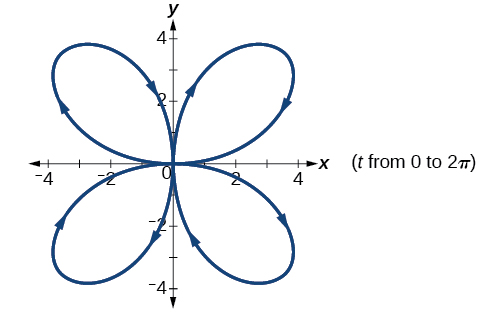(1+cosA+IsinA)(1+cosB+isinB)/(cos@+isin@)(cos$+isin$)
hatdog
Mark
how we can draw three triangles of distinctly different shapes. All the angles will be cutt off each triangle and placed side by side with vertices touching
bsc F. y algebra and trigonometry pepper 2
given that x= 3/5 find sin 3x
4
DB
remove any signs and collect terms of -2(8a-3b-c)
-16a+6b+2c
Will
Joeval
(x2-2x+8)-4(x2-3x+5)
sorry
Miranda
x²-2x+9-4x²+12x-20 -3x²+10x+11
Miranda
x²-2x+9-4x²+12x-20 -3x²+10x+11
Miranda
(X2-2X+8)-4(X2-3X+5)=0 ?
master
The anwser is imaginary number if you want to know The anwser of the expression you must arrange The expression and use quadratic formula To find the answer
master
The anwser is imaginary number if you want to know The anwser of the expression you must arrange The expression and use quadratic formula To find the answer
master
Y
master
master
Soo sorry (5±Root11* i)/3
master
Mukhtar
2x²-6x+1=0
Ife
explain and give four example of hyperbolic function
What is the correct rational algebraic expression of the given "a fraction whose denominator is 10 more than the numerator y?
y/y+10
Mr
Find nth derivative of eax sin (bx + c).
Find area common to the parabola y2 = 4ax and x2 = 4ay.
Anurag
y2=4ax= y=4ax/2. y=2ax
akash
A rectangular garden is 25ft wide. if its area is 1125ft, what is the length of the garden
to find the length I divide the area by the wide wich means 1125ft/25ft=45
Miranda
thanks
Jhovie
What do you call a relation where each element in the domain is related to only one value in the range by some rules?
A banana.
Yaona
given 4cot thither +3=0and 0°<thither <180° use a sketch to determine the value of the following a)cos thither
what are you up to?
nothing up todat yet
Miranda
hi
jai
hello
jai
Miranda Drice
jai
aap konsi country se ho
jai
which language is that
Miranda
I am living in india
jai
good
Miranda
what is the formula for calculating algebraic
I think the formula for calculating algebraic is the statement of the equality of two expression stimulate by a set of addition, multiplication, soustraction, division, raising to a power and extraction of Root. U believe by having those in the equation you will be in measure to calculate it
Miranda
state and prove Cayley hamilton therom
hello
Propessor
hi
Miranda
the Cayley hamilton Theorem state if A is a square matrix and if f(x) is its characterics polynomial then f(x)=0 in another ways evey square matrix is a root of its chatacteristics polynomial.
Miranda
hi
jai
hi Miranda
jai
thanks
Propessor
welcome
jai

#### Get Jobilize Job Search Mobile App in your pocket Now!ByByBy Janet ForresterBy Michael NelsonBy Cath YuBy JavaChamp TeamBy Abishek DevarajBy Mackenzie WilcoxBy Anh DaoBy Jonathan LongBy Jonathan LongBy Brooke Delaney